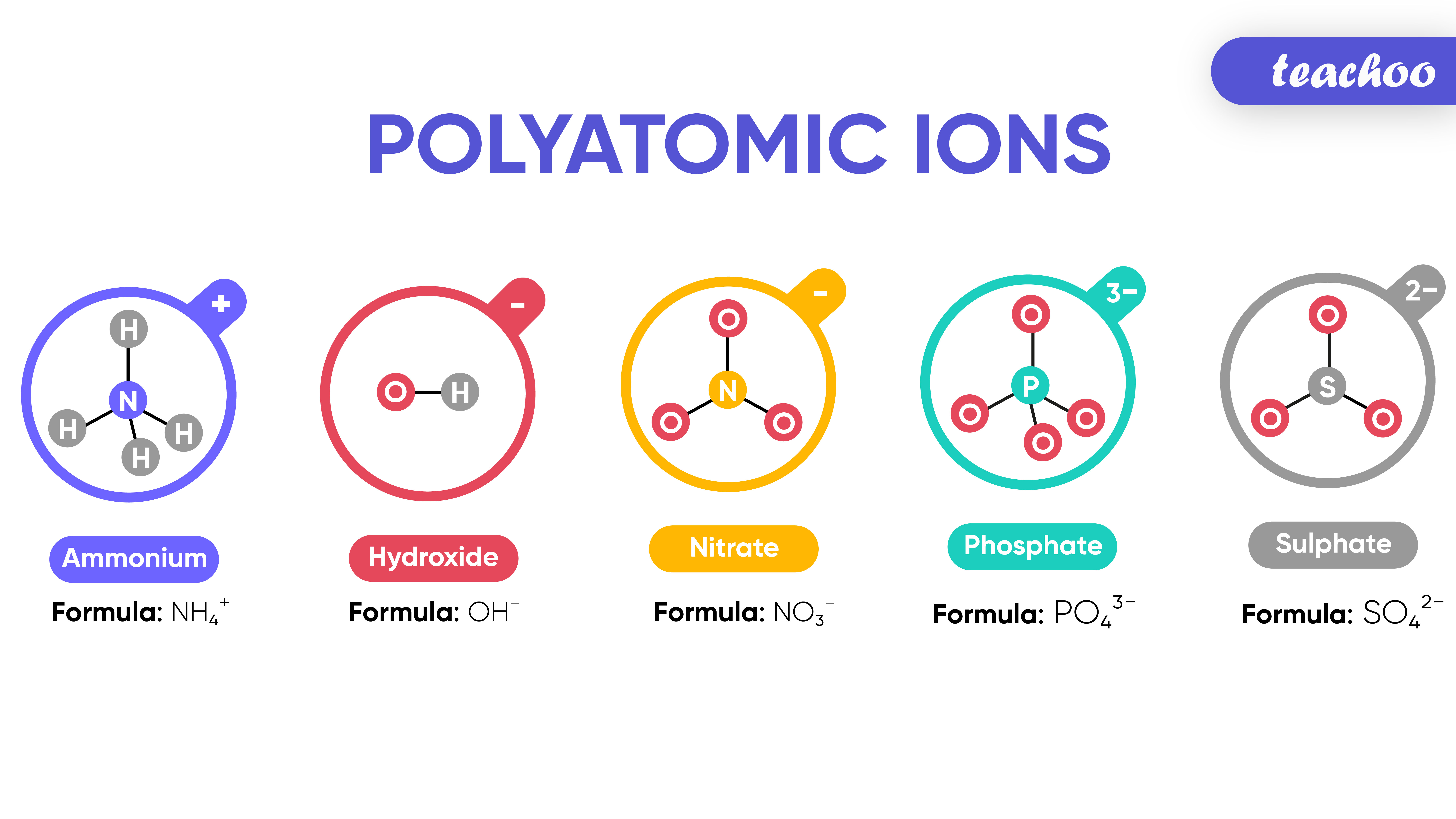‘Poly' means many

‘Atomic' means atoms

‘Ion' means atom carrying charged

Group of atoms containing charge is called Polyatomic Ion.## Examples of Polyatomic Ions

• Ammonium - NH 4 +

• Hydroxide - OH -

• Nitrate - NO 3 -

• Phosphate - PO 4 3-

• Sulphate - SO 4 2-

## What is a Molecule?

A group of atoms chemically bonded together is Here are the valency of a few elements:

called Molecule.

## How to write Formula of compounds formed with Polyatomic Ions

The formula of Polyatomic ion is written in brackets.

## Example - In Aluminium Sulphate Al 2 (SO 4 )3

SO 4 2- is a polyatomic ion consisting of Sulphur (S) and Oxygen (O)
The subscript ‘3’ in Al2(SO 4 ) 3 applies to both S and O
And so, SO 4 is put in brackets with subscript ‘3’ outside the brackets.
On the other hand, Al does not have brackets as it is monoatomic.

## Example - Magnesium Hydroxide Mg(OH)2

• Valency of Magnesium is 2
• Charge on Magnesium is 2+
• Charge on Hydroxide is 1-

These valencies interchange while writing formula as shown below

## What is a Molecule?

A group of atoms chemically bonded together is Here are the valency of a few elements:

called Molecule.

Hence Formula of Magnesium hydroxide is Mg(OH) 2

Note - Hydroxide is written in brackets as 2 is applicable to O and H which means there are 2 OH - ions (2 O and 2 H atoms)

## Example - Sodium Hydroxide NaOH

• Valency of Sodium is 1
• Charge on Sodium is 1+
• Charge on Hydroxide is 1-

These valencies interchange while writing formula as shown below

## What is a Molecule?

A group of atoms chemically bonded together is Here are the valency of a few elements:

called Molecule.

Hence Formula of Sodium hydroxide is NaOH

1. Class 9
2. Chapter 3 Class 9 - Atoms And Molecules (Term 2)
3. Concepts

Concepts

Class 9
Chapter 3 Class 9 - Atoms And Molecules (Term 2)